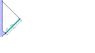# Solving Static Equilibrium Problem

• DrunkApple
In summary, the problem involves a uniform bar attached to a wall with a hinge and a cord at a 90 degree angle. The question asks for the magnitude of the horizontal force Hx on the pivot, with the acceleration of gravity given. The solution involves considering the two forces in the x direction acting on the bar and realizing that the force of gravity acts in the y direction. This can be solved by studying worked out examples and understanding the concepts.

#### DrunkApple

Static Equilibrium Problem

## Homework Statement

A uniform bar of length 16 m and mass 8 kg is
attached to a wall with a hinge that exerts a
horizontal force Hx and a vertical force Hy on
the bar. The bar is held by a cord that makes
a 90 degree angle with respect to the bar and 45 degree
with respect to the wall.
What is the magnitude of the horizontal
force Hx on the pivot? The acceleration of
gravity is 9.8 m/s2 .

## The Attempt at a Solution

I actually have no idea what I'm doing in this question. This chapter I do not get.

#### Attachments

•qwerty.png
6.9 KB · Views: 649
Last edited:
for Hx, why isn't x component of the rod included?

There are only two forces in the x direction that act on the bar, a piece of T and the force acting on the bar at the pivot I labeled F_x.

Good luck.

Yes yes but i don't understand why x component of Fg is left out though

What is Fg? Is that mg? If so mg acts in the y direction.

I got it. I got confused with this Fg because the rod was slanted and somehow mistaken it that the rod was on the incline -_- thank u mate :)